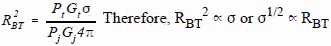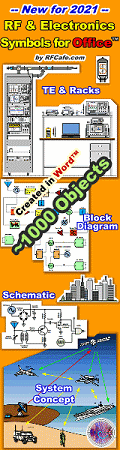About RF CafeCopyright: 1996 - 2024Webmaster:    Kirt Blattenberger,     BSEE - KB3UON RF Cafe began life in 1996 as "RF Tools" in an AOL screen name web space totaling 2 MB. Its primary purpose was to provide me with ready access to commonly needed formulas and reference material while performing my work as an RF system and circuit design engineer. The World Wide Web (Internet) was largely an unknown entity at the time and bandwidth was a scarce commodity. Dial-up modems blazed along at 14.4 kbps while tying up your telephone line, and a nice lady's voice announced "You've Got Mail" when a new message arrived... All trademarks, copyrights, patents, and other rights of ownership to images and text used on the RF Cafe website are hereby acknowledged. My Hobby Website: AirplanesAndRockets.com# Electronic Warfare and Radar Systems Engineering Handbook- Radar Cross Section (RCS) -

Radar cross section is the measure of a target's ability to reflect radar signals in the direction of the radar receiver, i.e. it is a measure of the ratio of backscatter power per steradian (unit solid angle) in the direction of the radar (from the target)
to the power density that is intercepted by the target.

The RCS of a target can be viewed as a comparison of the strength of the reflected signal from a target to the reflected signal from a perfectly smooth sphere of cross sectional area of 1 m2 as shown in Figure 1 .The conceptual definition of RCS includes the fact that not all of the radiated energy falls on the target. A target's RCS (σ) is most easily visualized as the product of three factors:

σ = Projected cross section x Reflectivity x Directivity .

RCS(σ) is used in Section 4-4 for an equation representing power reradiated from the target.

Reflectivity: The percent of intercepted power reradiated (scattered) by the target.

Directivity: The ratio of the power scattered back in the radar's direction to the power that would have been backscattered had the scattering been uniform in all directions (i.e. isotropically).Figures 2 and 3 show that RCS does not equal geometric area. For a sphere, the RCS, F = Br2, where r is the radius of the sphere.

The RCS of a sphere is independent of frequency if operating at sufficiently high frequencies where λ<<Range, and λ<< radius (r). Experimentally, radar return reflected from a target is compared to the radar return reflected from a sphere which has a frontal or projected area of one square meter (i.e. diameter of about 44 in). Using the spherical shape aids in field or laboratory measurements since orientation or positioning of the sphere will not affect radar reflection intensity measurements as a flat plate would. If calibrated, other sources (cylinder, flat plate, or corner reflector, etc.) could be used for comparative measurements.

To reduce drag during tests, towed spheres of 6", 14" or 22" diameter may be used instead of the larger 44" sphere, and the reference size is 0.018, 0.099 or 0.245 m2 respectively instead of 1 m2. When smaller sized spheres are used for tests you may be operating at or near where λ-radius. If the results are then scaled to a 1 m2 reference, there may be some perturbations due to creeping waves. See the discussion at the end of this section for further details.Figure 3. Backscatter From ShapesIn Figure 4, RCS patterns are shown as objects are rotated about their vertical axes (the arrows indicate the direction of the radar reflections).

The sphere is essentially the same in all directions.

The flat plate has almost no RCS except when aligned directly toward the radar.

The corner reflector has an RCS almost as high as the flat plate but over a wider angle, i.e., over ±60°. The return from a corner reflector is analogous to that of a flat plate always being perpendicular to your collocated transmitter and receiver.

Targets such as ships and aircraft often have many effective corners. Corners are sometimes used as calibration targets or as decoys, i.e. corner reflectors.

An aircraft target is very complex. It has a great many reflecting elements and shapes. The RCS of real aircraft must be measured. It varies significantly depending upon the direction of the illuminating radar.Figure 5 shows a typical RCS plot of a jet aircraft. The plot is an azimuth cut made at zero degrees elevation (on the aircraft horizon). Within the normal radar range of 3-18 GHz, the radar return of an aircraft in a given direction will vary by a few dB as frequency and polarization vary (the RCS may change by a factor of 2-5). It does not vary as much as the flat plate.

As shown in Figure 5, the RCS is highest at the aircraft beam due to the large physical area observed by the radar and perpendicular aspect (increasing reflectivity). The next highest RCS area is the nose/tail area, largely because of reflections off the engines or propellers. Most self-protection jammers cover a field of view of +/- 60 degrees about the aircraft nose and tail, thus the high RCS on the beam does not have coverage. Beam coverage is frequently not provided due to inadequate power available to cover all aircraft quadrants, and the side of an aircraft is theoretically exposed to a threat 30% of the time over the average of all scenarios.

Typical radar cross sections are as follows: Missile 0.5 sq m; Tactical Jet 5 to 100 sq m; Bomber 10 to 1000 sq m; and ships 3,000 to 1,000,000 sq m. RCS can also be expressed in decibels referenced to a square meter (dBsm) which equals 10 log (RCS in m2).

Again, Figure 5 shows that these values can vary dramatically. The strongest return depicted in the example is 100 m2 in the beam, and the weakest is slightly more than 1 m2 in the 135°/225° positions. These RCS values can be very misleading because other factors may affect the results. For example, phase differences, polarization, surface imperfections, and material type all greatly affect the results. In the above typical bomber example, the measured RCS may be much greater than 1000 square meters in certain circumstances (90°, 270°).

SIGNIFICANCE OF THE REDUCTION OF RCS

If each of the range or power equations that have an RCS (σ) term is evaluated for the significance of decreasing RCS, Figure 6 results. Therefore, an RCS reduction can increase aircraft survivability. The equations used in Figure 6 are as follows:

Range (radar detection): From the 2-way range equation in Section 4-4:Range (radar burn-through): The crossover equation in Section 4-8 has:Power (jammer): Equating the received signal return (Pr) in the two way range equation to the received jammer signal (Pr) in the one way range equation, the following relationship results:Therefore, Pjσ or σPj Note: jammer transmission line loss is combined with the jammer antenna gain to obtain Gt.Figure 6. Reduction of RCS Affects Radar Detection, Burn-through, and Jammer Power

Example of Effects of RCS Reduction - As shown in Figure 6, if the RCS of an aircraft is reduced to 0.75 (75%) of its original value, then (1) the jammer power required to achieve the same effectiveness would be 0.75 (75%) of the original value (or -1.25 dB). Likewise, (2) If Jammer power is held constant, then burn-through range is 0.87 (87%) of its original value (-1.25 dB), and (3) the detection range of the radar for the smaller RCS target (jamming not considered) is 0.93 (93%) of its original value (-1.25 dB).

OPTICAL / MIE / RAYLEIGH REGIONS

Figure 7 shows the different regions applicable for computing the RCS of a sphere. The optical region (“far field” counterpart) rules apply when 2Br/λ > 10. In this region, the RCS of a sphere is independent of frequency. Here, the RCS of a sphere, σ = πr2. The RCS equation breaks down primarily due to creeping waves in the area where λ-2πr. This area is known as the Mie or resonance region. If we were using a 6" diameter sphere, this frequency would be 0.6 GHz. (Any frequency ten times higher, or above 6 GHz, would give expected results). The largest positive perturbation (point A) occurs at exactly 0.6 GHz where the RCS would be 4 times higher than the RCS computed using the optical region formula. Just slightly above 0.6 GHz a minimum occurs (point B) and the actual RCS would be 0.26 times the value calculated by using the optical region formula. If we used a one meter diameter sphere, the perturbations would occur at 95 MHz, so any frequency above 950 MHz (-1 GHz) would give predicted results.

CREEPING WAVES

The initial RCS assumptions presume that we are operating in the optical region (λ<<Range and λ<<radius). There is a region where specular reflected (mirrored) waves combine with back scattered creeping waves both constructively and destructively as shown in Figure 8. Creeping waves are tangential to a smooth surface and follow the "shadow" region of the body. They occur when the circumference of the sphere - λ and typically add about 1 m2 to the RCS at certain frequencies.Figure 7. Radar Cross Section of a SphereFigure 8. Addition of Specular and Creeping WavesPlease Support RF Cafe by purchasing my  ridiculously low−priced products, all of which I created. These Are Available for Free